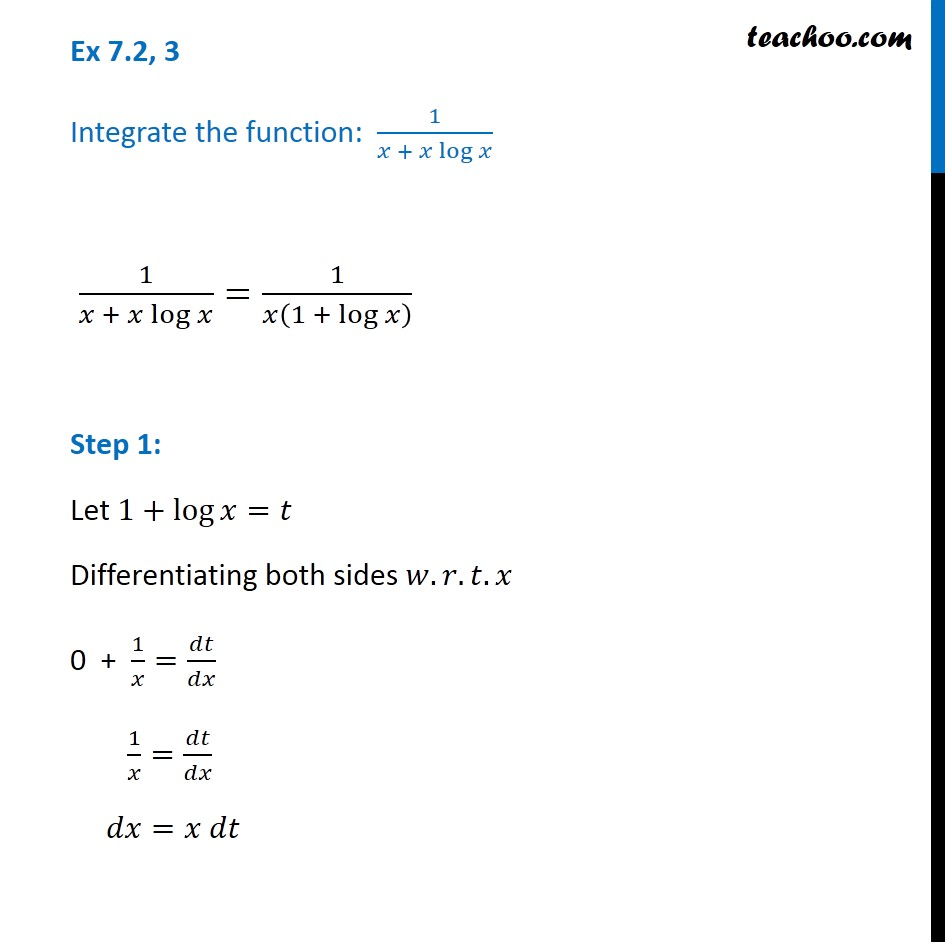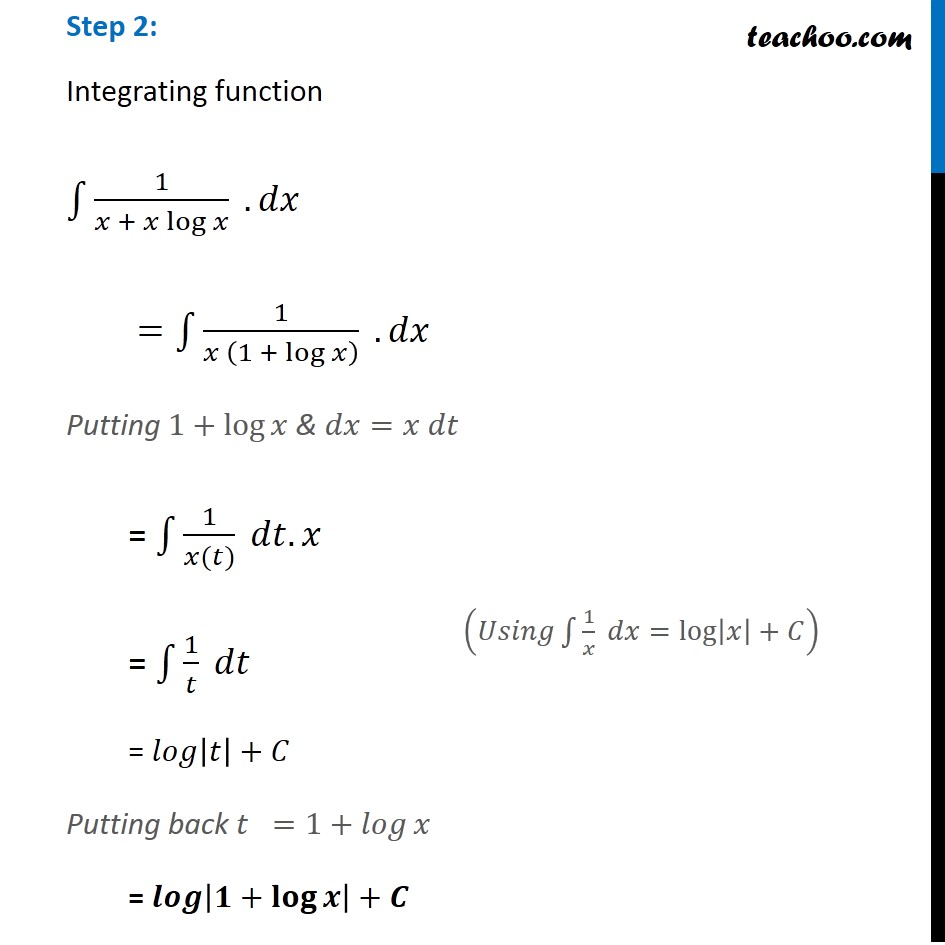Integration by substitution - lnx

Chapter 7 Class 12 Integrals
Concept wiseLearn in your speed, with individual attention - Teachoo Maths 1-on-1 Class

### Transcript

Ex 7.2, 3 Integrate the function: 1/(𝑥 + 𝑥 log⁡𝑥 ) 1/(𝑥 + 𝑥 log⁡𝑥 )=1/𝑥(1 + log⁡𝑥 ) Step 1: Let 1+log⁡𝑥=𝑡 Differentiating both sides 𝑤.𝑟.𝑡.𝑥 0 + 1/𝑥=𝑑𝑡/𝑑𝑥 1/𝑥=𝑑𝑡/𝑑𝑥 𝑑𝑥=𝑥 𝑑𝑡 Step 2: Integrating function ∫1▒1/(𝑥 + 𝑥 log⁡𝑥 ) .𝑑𝑥 =∫1▒1/(𝑥 (1 + log⁡𝑥 ) ) .𝑑𝑥" " Putting 1+log⁡𝑥 & 𝑑𝑥=𝑥 𝑑𝑡 = ∫1▒1/(𝑥(𝑡)) 𝑑𝑡.𝑥 = ∫1▒1/𝑡 𝑑𝑡 = 𝑙𝑜𝑔|𝑡|+𝐶 Putting back 𝑡 =1+𝑙𝑜𝑔⁡𝑥 = 𝒍𝒐𝒈|𝟏+𝐥𝐨𝐠⁡𝒙 |+𝑪 (𝑈𝑠𝑖𝑛𝑔∫1▒〖1/𝑥 𝑑𝑥〗=log⁡|𝑥|+𝐶)